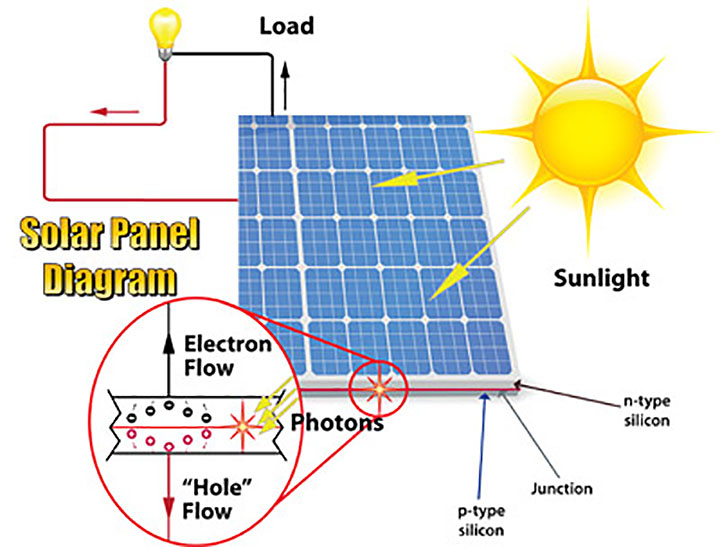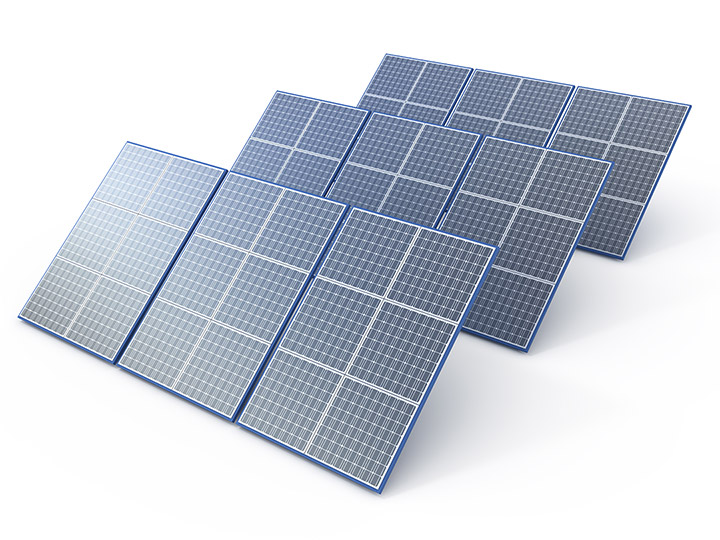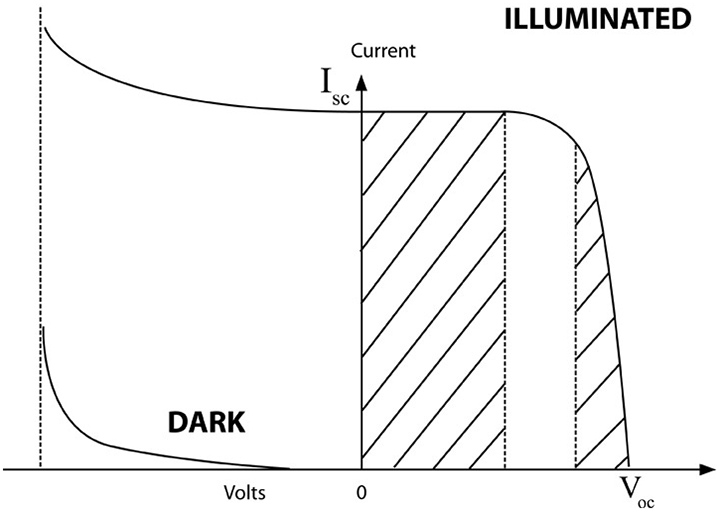# Photovoltaic Array Fundamentals

## Photovoltaic Array FundamentalsThe physics of the PV cell is very similar to the classical p-n junction diode. When light is absorbed by the junction, the energy of the absorbed photons is transferred to the electron system of the material, resulting in the creation of charge carriers that are separated at the junction. The charge carriers may be electron-ion pairs in a liquid electrolyte, or electron hole pairs in a solid semiconducting material. The charge carriers in the junction region create a potential gradient, get accelerated under the electric field and circulate as the current through an external circuit. The current squared times the resistance of the circuit is the power converted into electricity. The remaining power of the photon elevates the temperature of the cell.

A number of modules make up a typical Photovoltaic panel that can be connected in a string configuration in order to achieve desired current and voltage at the inverter input. A number of Photovoltaic panels connected in a string configuration is typically known as a Photovoltaic array.

Current versus voltage (I-V) characteristics of the PV module can be defined in sunlight and under dark conditions. In the first quadrant, the top left of the I-V curve at zero voltage is called the short circuit current. This is the current measured with the output terminals shorted (zero voltage). The bottom right of the curve at zero current is called the open-circuit voltage. This is the voltage measured with the output terminals open (zero current).If the voltage is externally applied in the reverse direction, for example, during a system fault transient, the current remains flat and the power is absorbed by the cell. However, beyond a certain negative voltage, the junction breaks down as in a diode, and the current rises to a high value. In the dark, the current is zero for voltage up to the breakdown voltage which is the same as in the illuminated condition.